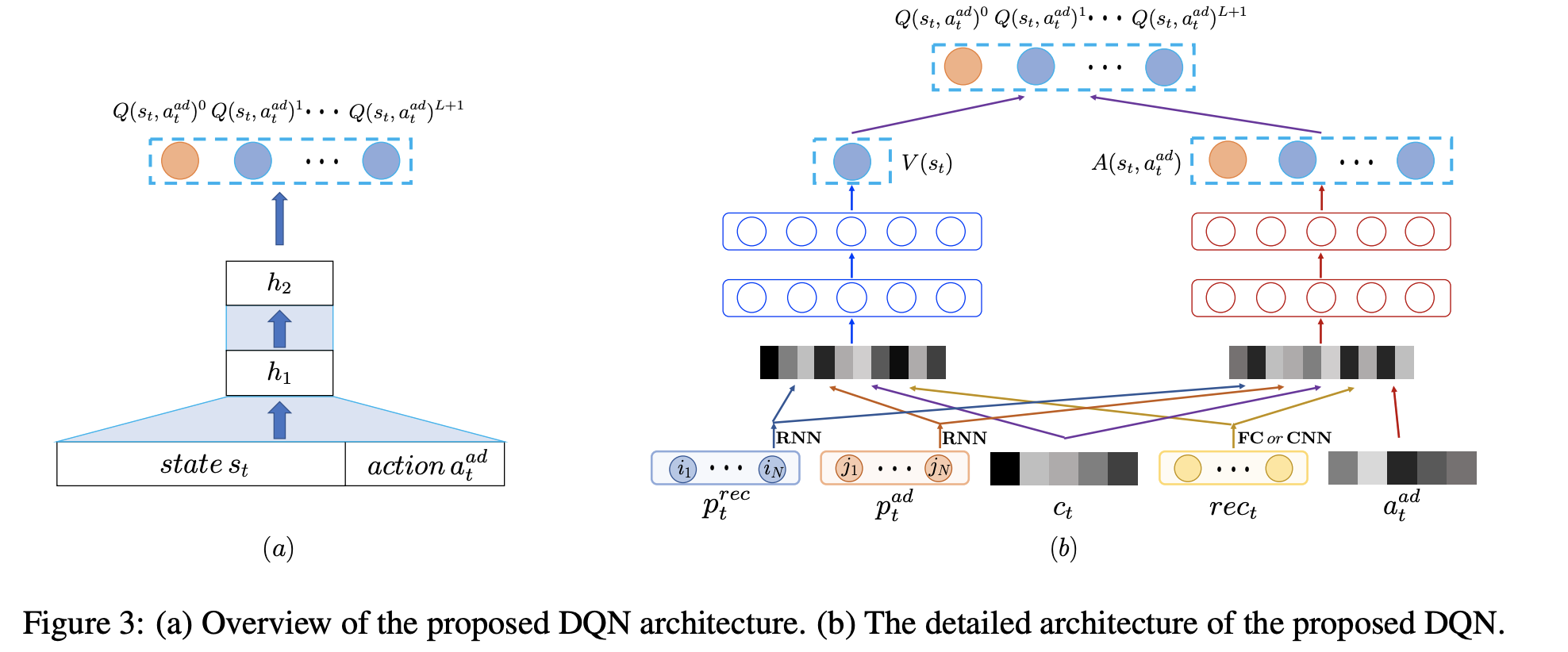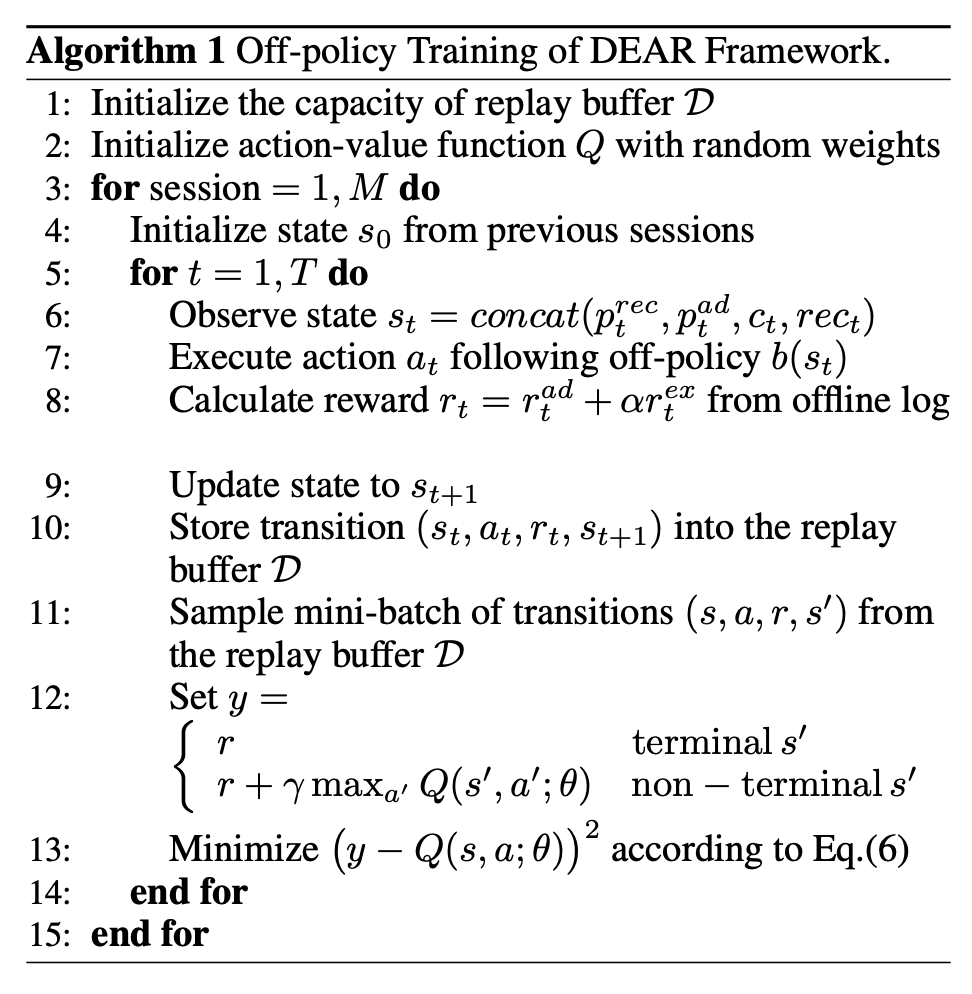To Top

## drn

https://daiwk.github.io/posts/rl-drn.html

## top-k off-policy

https://daiwk.github.io/posts/dl-topk-off-policy-correction.html

## slateq

https://daiwk.github.io/posts/rl-slateq.html

## DEAR

Deep Reinforcement Learning for Online Advertising in Recommender Systems

### dear模型结构

• 是否插入广告
• 如果插入，插入哪一条广告
• 插入广告在推荐列表的哪个位置

DQN的两种经典结构：

• 输入state，输出是所有可能action对应的Q
• 输入state以及某一个action，输出是对应的Q• state是把下面3部分concate到一起的一个向量：
• 使用GRU针对用户的推荐结果点击历史和广告点击历史进行用户序列偏好建模的$$p^{rec}_t$$$$p^{ad}_t$$
• 当前用户请求的上下文信息$$c$$，例如操作系统，app版本号，上下滑动等；
• 当前请求展示的推荐列表item的特征进行concat(当然也可以是cnn等)形成的低维稠密向量$$rec_{t}$$
• action包含两部分：
• 一部分是候选插入广告ad的特征$$a_t^{ad}$$，作为输入的一部分
• 另一部分则是广告插入的位置$$a_t^{loc}$$
• reward函数。Reward函数也包含两部分：
• 一部分是广告的的收入$$r^{ad}_t$$
• 另一部分则是用户是否继续往下刷的奖励$$r^{ex}_t$$，继续刷新是1，离开是-1。

$Q^{*}\left(s_{t}, a_{t}\right)=\mathbb{E}_{s_{t+1}}\left[r_{t}+\gamma \max _{a_{t+1}} Q^{*}\left(s_{t+1}, a_{t+1}\right) | s_{t}, a_{t}\right]$

• 针对用户请求构建状态$$s_t=concat(p^{rec}_t,p^{ad}_t,c_t,rec_t)$$
• 根据off-policy $$b(s_t)$$(即离线日志里的当前广告策略)执行action $$a_t$$，也就是选取特定ad；
• 更新状态为$$s_{t+1}$$
• 计算reward $$r_t=r_t^{ad}+\alpha r_t^{ex}$$
• 将状态转移信息$$(s_{t}，a_{t}，r_{t}，s_{t+1})$$存储到replay buffer；
• 从replay buffer中取出mini-batch的状态转移信息$$s,a,r,s'$$，来最小化$$(y-Q(s, a ; \theta))^{2}$$训练得到最优的Q函数参数。
• 其中，

$y=\left\{\begin{array}{ll}{r} & {\text { terminal } s^{\prime}} \\ {r+\gamma \max _{a^{\prime}} Q\left(s^{\prime}, a^{\prime} ; \theta\right)} & {\text { non - terminal } s^{\prime}}\end{array}\right.$• 得到状态$$s_t=concat(p^{rec}_t,p^{ad}_t,c_t,rec_t)$$
• 通过最优策略$$Q^*(s_t,a_t)$$执行动作$$a_t$$
• 得到用户反馈的reward $$r_t(s_t,a_t)$$
• 将状态从$$s_t$$更新到$$s_{t+1}$$### dear实验

100w的session，涉及到18w的user，1700w的自然结果视频，1000w的广告。

comment here..